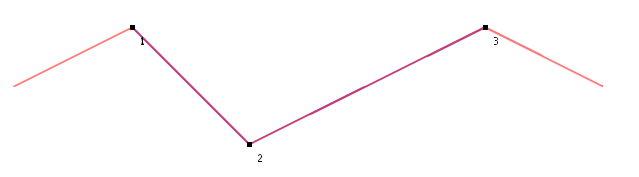2023.0.11

• All Known Implementing Classes:
TLcdCartesianBasicBinaryTopology, TLcdEllipsoidalBasicBinaryTopology, TLcdSphericalBasicBinaryTopology

`public interface ILcd2DLineIntersectionTopology`

This interface defines 2D outline intersection relations on `ILcdShape`s. Given two implementations of `ILcdShape`, it calculates the intersection points between the outlines of the given shapes.

### Calculation details

• For overlapping line segments, the first and last point of the overlapping segment are returned as intersection points.
• Single points do not line intersect any other shape.
• The result never contains any duplicate intersection points. Consider polygon ABC and polyline ABA. * The intersection point is only point A (once).

The line intersection operation always returns points. To get, for example, the intersection of two overlapping polygons as a polygon, you can use the constructive geometry framework instead.

### Examples

• Consider polygon ABC (A at the left of BC) and polygon BCD (D at the right of BC). The intersection points for these two polygons are B and C.
• Consider polygon ABC (A and B at the left of C) and polygon CDE (D and E at the right of C). The intersection point for these two polygons is C.
• Consider line segment AD, with points B and C lying on it. The intersection points between line AD and line BC are B and C.
• Consider two arcs that partially overlap each other. The start and end point of the overlapping part are returned as intersection points.
• For overlapping segments without a first or last point, such as overlapping circles and ellipses, no intersection points are returned.Intersection points of two polylines, where the blue polyline is shorter and lies on the red polyline

Since:
8.0
• ### Method Summary

All Methods
Modifier and Type Method and Description
`ILcdPoint[]` ```lineIntersection(ILcdShape aS1, ILcdShape aS2)```
Calculates the intersection points in 2D between the outlines of the given shapes.
`int` ```lineIntersectionSFCT(ILcdShape aS1, ILcdShape aS2, ILcd2DEditablePoint[] aPointsSFCT)```
Calculates the intersection points in 2D between the outlines of the given shapes.
• ### Method Detail

• #### lineIntersection

```ILcdPoint[] lineIntersection(ILcdShape aS1,
ILcdShape aS2)```
Calculates the intersection points in 2D between the outlines of the given shapes.

Note that the order of the intersection points might be unexpected.

Parameters:
`aS1` - The first shape.
`aS2` - The second shape.
Returns:
If both shapes intersect, an array of zero length if no intersection points were found (e.g., two overlapping circles) or an array containing the intersection points otherwise. If no intersection is found, `null` is returned.
Throws:
`IllegalArgumentException` - If either one of the shapes or its combination is not supported.
• #### lineIntersectionSFCT

```int lineIntersectionSFCT(ILcdShape aS1,
ILcdShape aS2,
ILcd2DEditablePoint[] aPointsSFCT)```
Calculates the intersection points in 2D between the outlines of the given shapes. The result is filled in the given point array. The number of intersection points is returned as an integer.

Note that the order of the intersection points might be unexpected.

Parameters:
`aS1` - The first shape.
`aS2` - The second shape.
`aPointsSFCT` - The array of points in which the results should be stored.
Returns:
If both shapes intersect, the number of intersection points that were found (>= 0; e.g., 0 for two overlapping circles). If no intersection is found, `-1` is returned.
Throws:
`IllegalArgumentException` - If either one of the shapes or its combination is not supported.
`IndexOutOfBoundsException` - If the length of `aPointsSFCT` is smaller than the number of intersection points.